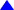ECON 6904                          SYLLABUS                                FALL 2004

INSTRUCTOR
: Ebenge Usip, Ph.D

OFFICE: 307 DeBartolo Hall; Hrs. Mon.-Th. 1-3, Th. 3-4; 742-1682

TEXT: Introductory Econometrics: A Modern Approach, 2ed, by Jeffrey M. Wooldridge
SUPPLEMENTS
(Available in the Department Conference Room)
Interpreting Mathematical Economics & Econometrics by B. D. Eastman
Fundamental Methods of Mathematical Economics (3rd ed.) by A. C. Chiang
Statistics for Business & Economics (9th ed.) by Anderson, Sweeney, & Williams (ASW)

• Exam # 1 (Date to be announced)                       20% of 500 points
• Exam # 2 (Date to be announced)                       20% of 500 points
• Homework                                                              10% of 500 points
• Final Exam (Dec. 9; 1800-2000)                          30% of 500 points
• APPROXIMATE SCALE: Will be discussed in class.
Note: the Last day to withdraw with a 'W'  is Oct. 23 (NOON)

OUTLINE
Part I: Fundamentals of Mathematical Economics - Functions, Differentiation, & Integration.

Functions: Applications in Modeling and Equilibrium/Static Analysis.
Eastman,
Preface thru ch. 2; Chiang, chs. 2 & 3
Differentiation: Applications in Comparative Static Analysis.
Eastman,
ch. 3; Chiang, chs. 6 (skip 6.5), 7 & 8

Applications in Optimization (Unconstrained & Constrained) Analysis.
Chiang
, chs. 9, & 12

Integration: Applications in Analysis of Economic Dynamics.
Eastman,
ch. 4; Chiang, ch. 13

Part II: Fundamentals of Mathematical Statistics
Introduction to Statistics, ASW, chs. 1 & 4
Probability and Probability Distributions,  ASW, chs. 5 & 6; Wooldridge, Appendix B.
Sampling & Sampling Distributions, ASW, chs. 7, 8, & 9; Wooldridge, Appendix C.
Statistical Inference: Interval Estimation & Hypothesis Testing

Part III: Fundamentals of Econometrics
Quantifying Economic Relationships. Eastman, ch. 5.
Regression Analysis (The Heart of Econometrics) ASW, chs. 14, & 15.
The nature of Econometrics & Economic Data. Wooldridge, ch. 1.

NOTES:
1. No make-up exams will be administered. Late homework will not be graded.
2. It is the student's responsibility to be familiar with the assigned readings and all lecture materials.

GOAL OF THE COURSE

In this course, we will study the quantitative methods that economists use to develop/construct/formulate economic theories and to quantify/measure economic relationships that derive from theoretical models/formulations. Developing economic theories rely heavily on mathematical concepts and techniques while quantifying economic relationships rely heavily on statistical concepts and techniques.

Applications of mathematical and statistical fundamentals will cover many areas of economics including:
● Microeconomics (e.g., individual consumer’s utility maximization, individual firm’s profit maximization, competition between firms, static and dynamic analysis of markets).
● Macroeconomics (e.g., the Classical and Keynesian theories of income determination).
● Monetary Economics (e.g., the Quantity theory of money; interest rate theories, and growth theories)
● International Economics (e.g., the theory of comparative advantage as the basis for  mutual gains from trade)
● Econometrics: A highly specialized field that provides the methodology for measuring economic relationships and testing hypotheses about the underlying theories.

During the course of this semester, for instance, many microeconomic topics will require mathematical rigor involving the calculus techniques of differentiation and integration. To use these techniques, you must first be familiar with the basic concepts of sets, variables, relations, and functions. You also must be able to distinguish between equations that stipulate/represent “definitional” relationships, “behavioral” relationships, and “equilibrium” relationships.

Our main aim is to expose you to only those mathematical/statistical techniques and symbols that are essential for understanding journal articles and for undertaking a serious applied economic analysis. We will not examine advanced topics such as matrix algebra which theoretical economists use extensively to arrive at sophisticated results; for instance, they employ the “Cramer’s” rule to solve a system of behavioral equations in matrix form to obtain equilibrium solutions.Top or Return to Course Information or Home Page.